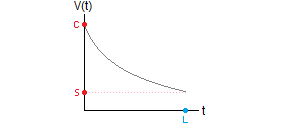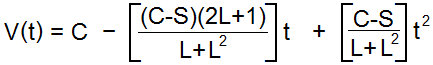# How to Calculate Sum-of-Years Depreciation

Sum-of-Years Depreciation CalculatorPurchase Price \$
Scrap Value \$
Lifespan      years

Sum-of-years, (aka SOY or sum of the years' digits) is a method of figuring asset depreciation. Like double declining balance depreciation, it is a method of accelerated depreciation that assumes an object loses more of its value in the early part of its useful life. It predicts more rapid decrease in value than straight line depreciation, but less rapid than double declining balance or exponential depreciation.

To compute the sum-of-years depreciation, you must first compute the sum of the years in the lifespan of the object, and then use that number to compute the percent decrease for each year. The example below will show you how to calculate the asset's diminished value at the end of each year. Or, you can use the calculator on the left to find an explicit formula for the object's worth.

### Sum of the Years' Digits Method

If the useful life of the object is L years, then first compute the sum of the all the numbers between 1 and L. For example, if a server lasts 8 years, then the sum of the years is

1+2+3+4+5+6+7+8 = 36.

Next, compute the total loss of value over the lifespan of the object. For example, if the server costs \$6000 and its scrap value is \$600, then the total loss is \$6000 - \$600 = \$5400. Now determine each years' depreciation using the following pattern:

 Year 1 Loss = (8/36)(5400) = \$1200 Year 2 Loss = (7/36)(5400) = \$1050 Year 3 Loss = (6/36)(5400) = \$900 Year 4 Loss = (5/36)(5400) = \$750 Year 5 Loss = (4/36)(5400) = \$600 Year 6 Loss = (3/36)(5400) = \$450 Year 7 Loss = (2/36)(5400) = \$300 Year 8 Loss = (1/36)(5400) = \$150

For this method, you take the year divided by the sum of the years (in reverse order) to determine the ratio of loss for each year. Putting it all together, the value at the end of each year is

 Year 1 End of Year Value = 6000 - 1200 = \$4800 Year 2 End of Year Value = 4800 - 1050 = \$3750 Year 3 End of Year Value = 3750 - 900 = \$2850 Year 4 End of Year Value = 2850 - 750 = \$2100 Year 5 End of Year Value = 2100 - 600 = \$1500 Year 6 End of Year Value = 1500 - 450 = \$1050 Year 7 End of Year Value = 1050 - 300 = \$750 Year 8 End of Year Value = 750 - 150 = \$600

You can also find an explicit equation V(t) for the value at the end of year t. Call C the original price, S the scrap value, and L the lifespan. Then the equation isUsing the example above, the value equation for the server is

V(t) = 6000 - 1275t + 75t2.